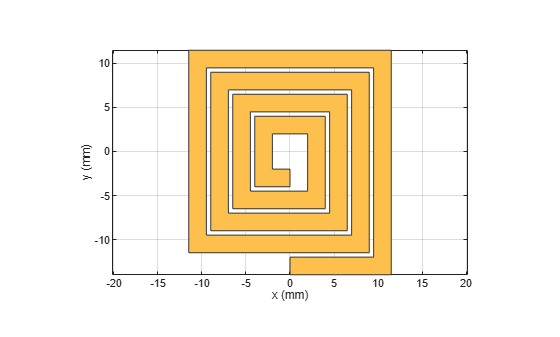# rotateX

Rotate RF PCB shape about x-axis

## Syntax

``c = rotateX(shape,angle)``

## Description

example

````c = rotateX(shape,angle)` rotates a shape by the specified angle about the x-axis.```

## Examples

collapse all

Create and display a spiral trace.

```trace = traceSpiral; show(trace)```Rotate the spiral trace by 45 degrees about the x-axis.

`traceRotX = rotateX(trace,45);`

Display the rotated shape. Set the camera line of sight to display in 3-D space.

```show(traceRotX) view(60,10)```## Input Arguments

collapse all

RF PCB shape created using custom elements and shape objects of RF PCB Toolbox™, specified as an object.

Example: `shape = bendCurved;` specifies the shape as a `bendCurved` object.

Angle of rotation, specified as a scalar in degrees.

Example: `45` rotates the shape around the x-axis by 45 degrees.

Data Types: `double`

## Version History

Introduced in R2021b